Examples

Chapter 1 Class 10 Real Numbers
Serial order wise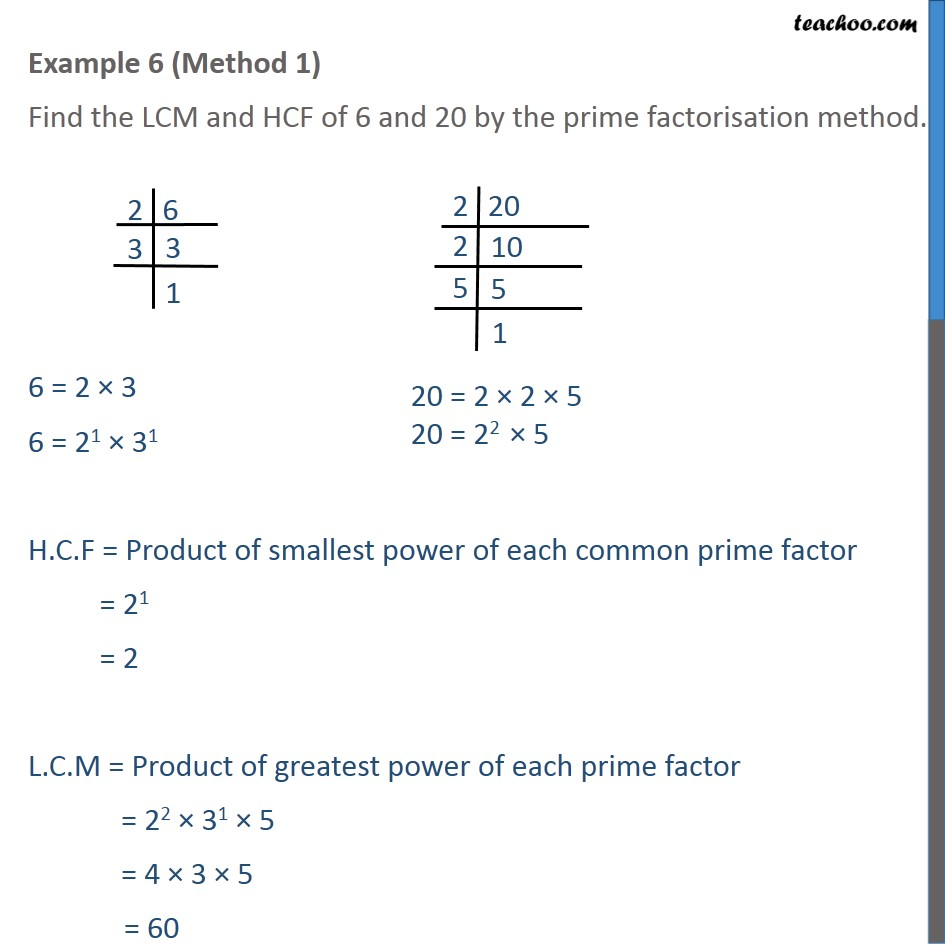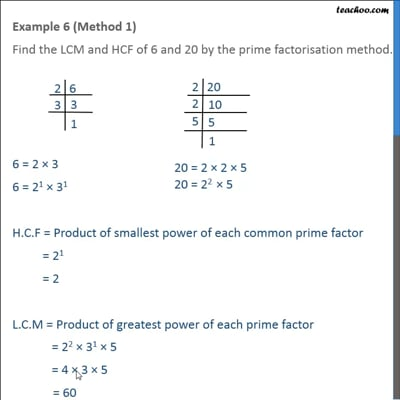This video is only available for Teachoo black users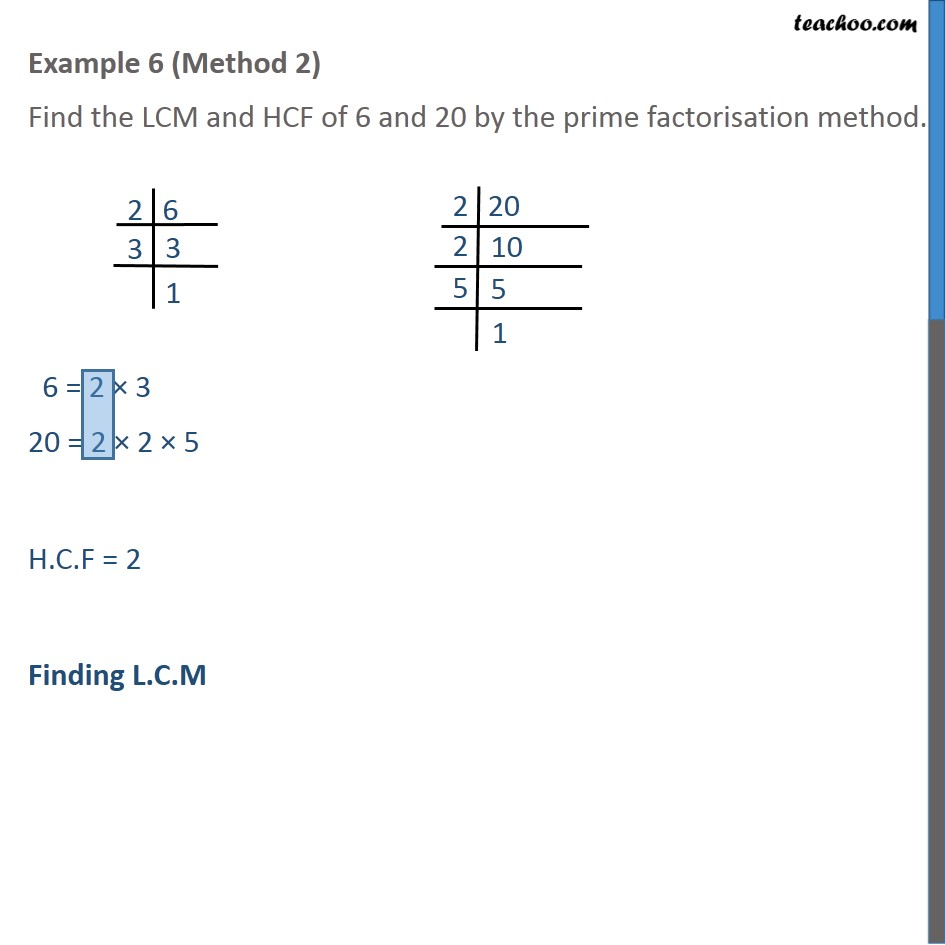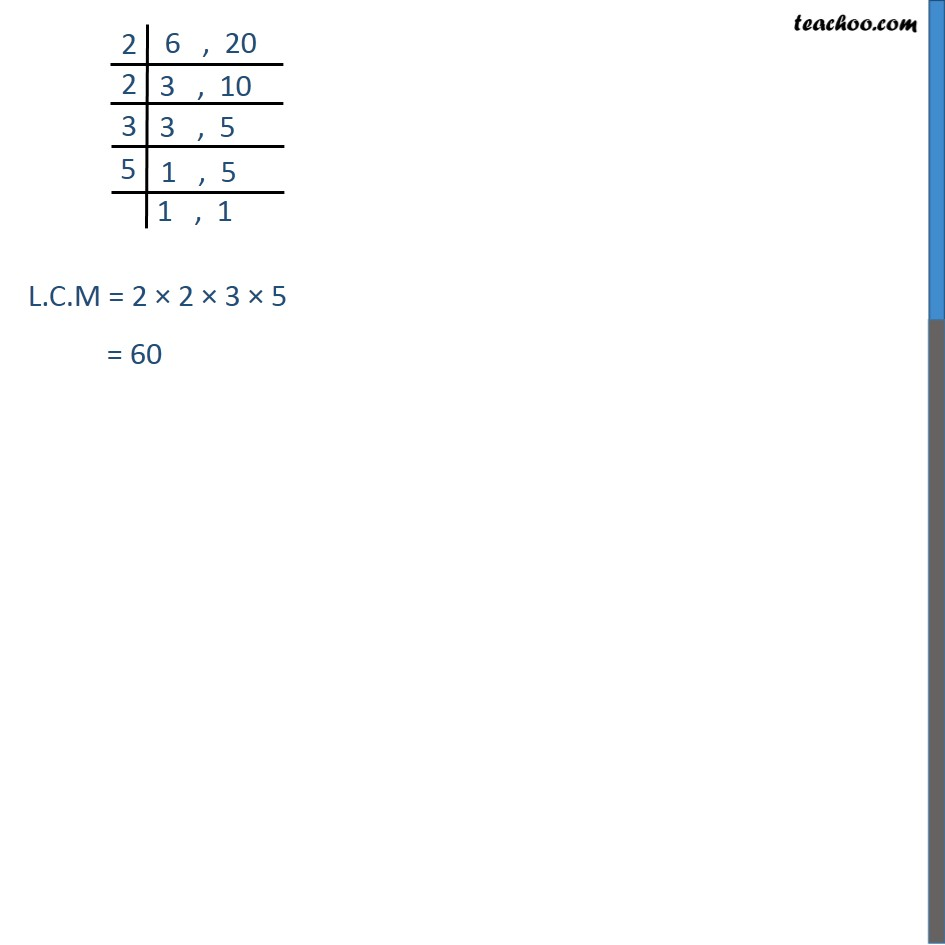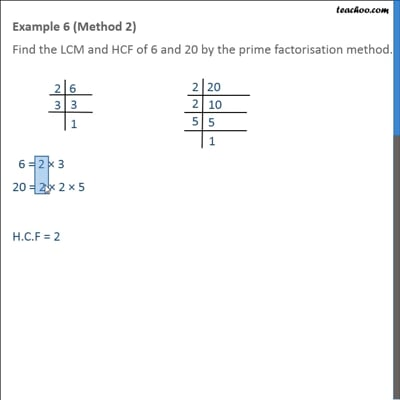This video is only available for Teachoo black users

Solve all your doubts with Teachoo Black (new monthly pack available now!)

### Transcript

Example 6 (Method 1) Find the LCM and HCF of 6 and 20 by the prime factorisation method. 6 = 2 3 6 = 21 31 H.C.F = Product of smallest power of each common prime factor = 21 = 2 L.C.M = Product of greatest power of each prime factor = 22 31 5 = 4 3 5 = 60 Example 6 (Method 2) Find the LCM and HCF of 6 and 20 by the prime factorisation method. 6 = 2 3 20 = 2 2 5 H.C.F = 2 Finding L.C.M L.C.M = 2 2 3 5 = 60